`Packages  This Package  Prev  Next  Index  `

# §1.10 Class Math

```public  final  class  java.lang.Math
extends  java.lang.Object  (I-§1.12)
{
// Fields
public final static double E;	§1.10.1
public final static double PI;	§1.10.2

// Methods
public static double abs(double  a);	§1.10.3
public static float abs(float  a);	§1.10.4
public static int abs(int  a);	§1.10.5
public static long abs(long  a);	§1.10.6
public static double acos(double  a);	§1.10.7
public static double asin(double  a);	§1.10.8
public static double atan(double  a);	§1.10.9
public static double atan2(double  a, double  b);	§1.10.10
public static double ceil(double  a);	§1.10.11
public static double cos(double  a);	§1.10.12
public static double exp(double  a);	§1.10.13
public static double floor(double  a);	§1.10.14
public static double                        §1.10.15
IEEEremainder(double  f1, double  f2);
public static double log(double  a);	§1.10.16
public static double max(double  a, double  b);	§1.10.17
public static float max(float  a, float  b);	§1.10.18
public static int max(int  a, int  b);	§1.10.19
public static long max(long  a, long  b);	§1.10.20
public static double min(double  a, double  b); 	§1.10.21
public static float min(float  a, float  b);	§1.10.22
public static int min(int  a, int  b);	§1.10.23
public static long min(long  a, long  b);	§1.10.24
public static double pow(double  a, double  b);	§1.10.25
public static double random();	§1.10.26
public static double rint(double  a);	§1.10.27
public static long round(double  a);	§1.10.28
public static int round(float  a);	§1.10.29
public static double sin(double  a);	§1.10.30
public static double sqrt(double  a);	§1.10.31
public static double tan(double  a);	§1.10.32
}
```
The class Math contains methods for performing basic numerical operations such as the elementary exponential, logarithm, square root, and trigononetric functions.

To help insure portability of Java programs, the definitions of many of the numerical functions in this package require that they produce the same results as certain published algorithms. These algorithms are available from the well-known network library netlib as the package fdlibm ("Freely Distributable Math Library"). These algorithms, which are written in the C programming language, are then to understood as executed with all floating-point operations following the rules of Java floating-point arithmetic.

The network library may be found on the World Wide Web at
http://netlib.att.com
then perform a keyword search for fdlibm.

The Java math library is defined with respect to the version of fdlibm dated 95/01/04. Where fdlibm provides more than one definition for a function (such as acos), the "IEEE754 core function" version is to be used (residing in a file whose name begins with the letter e).

##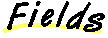### E

```public final static double E = 2.7182818284590452354 ```
The double value that is closer than any other to e, the base of the natural logarithms.

### PI

```public final static double PI = 3.14159265358979323846 ```
The double value that is closer than any other to, the ratio of the circumference of a circle to its diameter.

##### abs

```public static double abs(double a) ```
Calculates the absolute value of the argument.
Parameters:
```a - ```a double value
Returns:
the absolute value of the argument1.

### abs

```public static float abs(float a) ```
Calculates the absolute value of the argument.
Parameters:
```a - ```
a float value
Returns:
the absolute value of the argument2.

### abs

```public static int abs(int a) ```
Calculates the absolute value of the argument. If the argument is not negative, the argument is returned. If the argument is negative, the negation of the argument is returned.
Note that if the argument is equal to the value of Integer.MIN_VALUE (I-§1.8.2), the most negative representable int value, the result is that same value, which is negative.

Parameters:
```a - ```
an int value
Returns:
the absolute value of the argument.

### abs

```public static long abs(long a) ```
Calculates the absolute value of the argument.If the argument is not negative, the argument is returned. If the argument is negative, the negation of the argument is returned.
Note that if the argument is equal to the value of Long.MIN_VALUE (I-§1.9.2), the most negative representable long value, the result is that same value, which is negative.

Parameters:
```a - ```a long value.
Returns:
the absolute value of the argument.

### acos

```public static double acos(double a) ```
Parameters:
```a - ```a double value
Returns:
the arc cosine of the argument.

### asin

```public static double asin(double a) ```
Parameters:
```a - ```a double value
Returns:
the arc sine of the argument.

### atan

```public static double atan(double a) ```
Parameters:
```a - ```a double value
Returns:
the arc tangent of the argument.

### atan2

```public static double atan2(double a, double b) ```
Parameters:
```a - ```a double value
```b - ```a double value
Returns:
thecomponent of the polar coordinate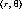that corresponds to the cartesian coordinate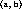.

### ceil

```public static double ceil(double a) ```
Returns the smallest (closest to negative infinity) double value that is not less than the argument and is equal to a mathematical integer.
Parameters:
```a - ```a double value
Returns:
the value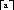.

### cos

```public static double cos(double a) ```
Parameters:
```a - ```an angle, in radians.
Returns:
the cosine of the argument

### exp

```public static double exp(double a) ```
Parameters:
```a - ```a double value
Returns:
the value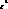, where where e (I-§1.10.1) is the base of the natural logarithms.

### floor

```public static double floor(double a) ```
Returns the largest (closest to positive infinity) double value that is not greater than the argument and is equal to a mathematical integer.
Parameters:
```a - ```a double value
Parameters:
```a - ```an assigned value
Returns:
the value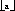.

### IEEEremainder

```public static double IEEEremainder(double f1, double f2) ```
Computes the remainder operation on two arguments as prescribed by the IEEE 754 standard: the remainder value is mathematically equal towhereis the mathematical integer closest to the exact mathematical value of the quotient, and if two mathematical integers are equally close tothenis the integer that is even. If the remainder is zero, its sign is the same as the sign of the first argument.
Parameters:
```f1 - ```the dividend
```f2 - ```the divisor
Returns: the remainder when f1 is divided by f2.

### log

`public static double log(double a)````3 ```
Parameters:
```a - ```a number greater than 0.0
Returns:
the value, the natural logarithm of a.

### max

```public static double max(double a, double b) ```
Parameters:
```a - ```a double value
```b - ```a double value
Returns:
the larger of a and b4.

### max

```public static float max(float a, float b) ```
Parameters:
```a - ```a float value
```b - ```a float value
Returns:
the larger of a and b5.

### max

```public static int max(int a, int b) ```
Parameters:
```a - ```an int value
```b - ```an int value
Returns:
the larger of a and b.

### max

```public static long max(long a, long b) ```
Parameters:
```a - ```a long value
```b - ```a long value
Returns:
the larger of a and b.

### min

```public static double min(double a, double b) ```
Parameters:
```a - ```a double value
```b - ```a double value
Returns:
the smaller of a and b6.

### min

```public static float min(float a, float b) ```
Parameters:
```a - ```a float value
```b - ```a float value
Returns:
the smaller of a and b7.

### min

```public static int min(int a, int b) ```
Parameters:
```a - ```an int value
```b - ```an int value
Returns:
the smaller of a and b.

### min

```public static long min(long a, long b) ```
Parameters:
```a - ```a long value
```b - ```a long value
Returns:
the smaller of a and b.

### pow

`public static double pow(double a, double b)````8 ```
Parameters:
```a - ```a double value
```b - ```a double value
Returns:
the value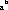.

### random

```public static double random() ```
Returns:
a pseudorandom double between 0.0 and 1.0.
nextDouble in class Random (I-§3.7.3).

### rint

```public static double rint(double a) ```
Calculates the closest integer to the argument.
Parameters:
```a - ```a double value
Returns:
the closest double value to a that is equal to a mathematical integer. If two double values that are mathematical integers are equally close to the value of the argument, the result is the integer value that is even.

### round

```public static long round(double a) ```
Calculates the closest long to the argument.
If the argument is negative infinity or any value less than or equal to the value of Long.MIN_VALUE (I-§1.9.2), the result is equal to the value of Long.MIN_VALUE.

If the argument is positive infinity or any value greater than or equal to the value of Long.MAX_VALUE (I-§1.9.1), the result is equal to the value of Long.MAX_VALUE.

Parameters:
```a - ```a double value
Returns:
the value of the argument rounded to the nearest long value.

### round

```public static int round(float a) ```
Calculates the closest int to the argument.
If the argument is negative infinity or any value less than or equal to the value of Integer.MIN_VALUE (I-§1.8.2), the result is equal to the value of Integer.MIN_VALUE.

If the argument is positive infinity or any value greater than or equal to the value of Integer.MAX_VALUE (I-§1.8.1), the result is equal to the value of Integer.MAX_VALUE.

Parameters:
```a - ```a float value
Returns:
the value of the argument rounded to the nearest int value.

### sin

```public static double sin(double a) ```
Parameters:
```a - ```a double value
Returns:
the sine of the argument

### sqrt

`public static double sqrt(double a)````9 ```
Parameters:
```a - ```a double value
Returns:
the value of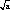. If the argument is NaN or less than zero, the result is NaN.

### tan

```public static double tan(double a) ```
Parameters:
```a - ```a double value
Returns:
the tangent of the argument.

1 In Java 1.0, abs(-0.0) returns -0.0. This bug is fixed in Java 1.1.

2 In Java 1.0, abs(-0.0f) returns -0.0f. This bug is fixed in Java 1.1.

3 In Java 1.0, the method log was declared as follows

4 In Java 1.0, max(-0.0, 0.0) returns -0.0. This bug is fixed in Java 1.1.

5 In Java 1.0, max(-0.0f, 0.0f) returns -0.0f. This bug is fixed in Java 1.1.

6 In Java 1.0, min(0.0, -0.0) returns 0.0. This bug is fixed in Java 1.1.

7 In Java 1.0, min(0.0f, -0.0f) returns 0.0f. This bug is fixed in Java 1.1.

8 In Java 1.0, the method pow was declared as follows

9 In Java 1.0, the method sqrt was declared as follows

`Packages  This Package  Prev  Next  Index`
Java API Document (HTML generated by dkramer on April 22, 1996)